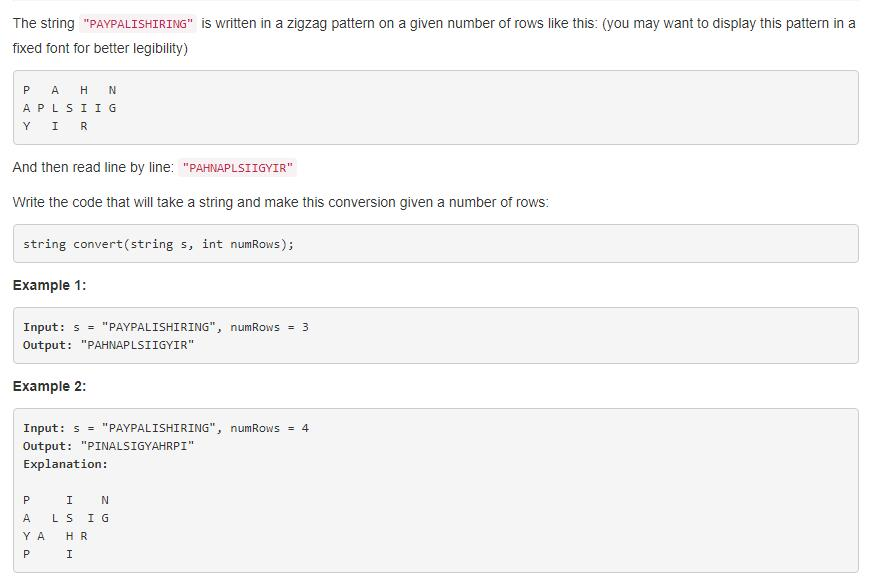# 每日算法系列【LeetCode 239】滑动窗口最大值

+关注继续查看

## 题目描述

输入
：nums = [1,3,-1,-3,5,3,6,7], 和 k = 3

-----[1  3  -1] -3  5  3  6  7
3 1 [3  -1  -3] 5  3  6  7
3 1  3 [-1  -3  5] 3  6  7
5 1  3  -1 [-3  5  3] 6  7
5 1  3  -1  -3 [5  3  6] 7
6 1  3  -1  -3  5 [3  6  7]
7

## 题解

### 双端单调队列

• 遍历元素 nums[i] ，然后跟队列尾部元素比较，如果比尾部元素大，就出队，然后继续比较，直到 nums[i] 小于尾部元素，然后将它入队。
• 然后用一下队列首部元素的下标，计算出队列中区间的长度，如果大于 k 了，那么队首元素就要出队。
• 最后队首元素就是当前区间的最大值。

## 代码

### 双端单调队列（c++）

class Solution {
public:
vector<int> maxSlidingWindow(vector<int>& nums, int k){
int n = nums.size();
deque<int> Q;
vector<int> res;
for (int i = 0; i < n; ++i) {
while (!Q.empty() && nums[i] >= nums[Q.back()])
Q.pop_back();
Q.push_back(i);
if (i - Q.front() + 1 > k) Q.pop_front();
if (i >= k-1) res.push_back(nums[Q.front()]);
}        return res;
}
};

### 双端单调队列+数组实现（c++）

class Solution {
public:

vector<int> maxSlidingWindow(vector<int>& nums, int k) {
int n = nums.size();
vector<int> Q(n, 0);
vector<int> res;
int l = 0, r = 0;
for (int i = 0; i < n; ++i) {
while (r-l > 0 && nums[i] >= nums[Q[r-1]]) r--;
Q[r++] = i;
if (i - Q[l] + 1 > k) l++;
if (i >= k-1) res.push_back(nums[Q[l]]);
}
return res;
}
};

### 分块法（c++）

class Solution {
public:
vector<int> maxSlidingWindow(vector<int>& nums, int k) {
int n = nums.size();
vector<int> lmax(n, 0), rmax(n, 0);
vector<int> res;
if (!n) return res;
for (int i = 0; i < n; ++i) {
if (i%k == 0) lmax[i] = nums[i];
else lmax[i] = max(lmax[i-1], nums[i]);
}
for (int i = n-1; i >= 0; --i) {
if ((i+1)%k|| i == n-1) rmax[i] = nums[i];            else rmax[i] = max(rmax[i+1], nums[i]);
}
for (int i = k-1; i < n; ++i) {
res.push_back(max(lmax[i], rmax[i-k+1]));
}
return res;
}
};

### 双端单调队列（python）

import collections
class Solution:
def maxSlidingWindow(self, nums: List[int], k: int) -> List[int]:
n = len(nums)
Q = collections.deque()
res = []
for i in range(n):
while len(Q) > 0 and nums[i] >= nums[Q[-1]]:
Q.pop()
Q.append(i)
if i - Q + 1 > k:
Q.popleft()
if i >= k-1:
res.append(nums[Q])
return res

### 双端单调队列+数组实现（python）

import collections
class Solution:
def maxSlidingWindow(self, nums: List[int], k: int) -> List[int]:
n = len(nums)
Q =  * n
res = []
l, r = 0, 0
for i in range(n):
while r-l > 0 and nums[i] >= nums[Q[r-1]]:
r -= 1
Q[r] = i
r += 1
if i - Q[l] + 1 > k:
l += 1
if i >= k-1:
res.append(nums[Q[l]])
return res

### 分块法（python）

import collections
class Solution:
def maxSlidingWindow(self, nums: List[int], k: int) -> List[int]:
n = len(nums)
lmax, rmax =  * n,  * n
res = []
if n == 0:
return res
for i in range(n):
if i%k == 0:
lmax[i] = nums[i]
else:
lmax[i] = max(lmax[i-1], nums[i])
for i in range(n-1, -1, -1):
if (i+1)%k == 0 or i == n-1:
rmax[i] = nums[i]
else:
rmax[i] = max(rmax[i+1], nums[i])
for i in range(k-1, n):
res.append(max(lmax[i], rm)        return res


### 后记LeetCode 6-10 题 详解 Java版 ( 万字 图文详解 LeetCode 算法题6-10 =====＞＞＞ ＜建议收藏＞)
LeetCode 6-10 题 详解 Java版 ( 万字 图文详解 LeetCode 算法题6-10 =====＞＞＞ ＜建议收藏＞)
12 028 01023 0JavaScript-----------基本包装类型
839 0JavaScript-----------基本包装类型
837 01523 0
217

0